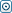Forex Stock Exchange Forum
Would you like to react to this message? Create an account in a few clicks or log in to continue.

# Calculating #Forex Profit and Losstime is money
Number of messages : 489
Points : 3010
Date of Entry : 2015-04-03
Year : 30

##Calculating #Forex Profit and Loss

on Fri Jun 12, 2015 7:29 pm
In order to make a profit on a trade, you need to ‘beat the spread’ – meaning that the price has to move in your favour by more than the difference between the two prices in order for you to turn a profit. Here, we shall show you how to calculate your profit or loss, using pip values and leverage amounts.

For example, let’s say you want to ‘buy’ USD/CHF, which means that you are effectively buying US dollars and selling Swiss francs. The price is quoted as follows:

USD/CHF = 1.4525 / 1.4530

Because you are buying US dollars, you will be working with the ‘ask’ price on the right. Let’s say you buy one standard lot (100,000 units) at 1.4530. An hour later, the price is quoted as follows:

USD/CHF = 1.4550 / 1.4555

As you can see, the spread (the difference between the bid and ask price) is still 5 pips. Because you are now selling USD in order to close the trade, you will be working with the ‘bid’ price on the left. The difference between the earlier ‘ask’ price (1.4530) and the current ‘bid’ price (1.4550) is 20 pips, or 0.0020. Your profit or loss can be calculated using the following equation:

Pip value (0.0001 x bid price) x Lot size x Change in pip value = profit or loss
(0.0001 x 1.4550 = 0.0001455) x 100,000 x 20 = 291 USD

However, if the trade had gone the other way, and the bid price had moved to 1.4510, the change in pip value (earlier ask price 1.4530 minus current bid price 1.4510) would have been –20, which means you would have made a loss of 291 USD. Here is the equation that demonstrates this:

Pip value (0.0001 x bid price) x Lot size x Change in pip value = profit or loss
(0.0001x 1.4550 = 0.0001455) x 100,000 x -20 = -291 USD

Remember that these equations only apply when you are buying and selling the base currency. If you are buying and selling the quote currency, you have to replace the bid price with the ask price in each of the equations.
Permissions in this forum:
You cannot reply to topics in this forum